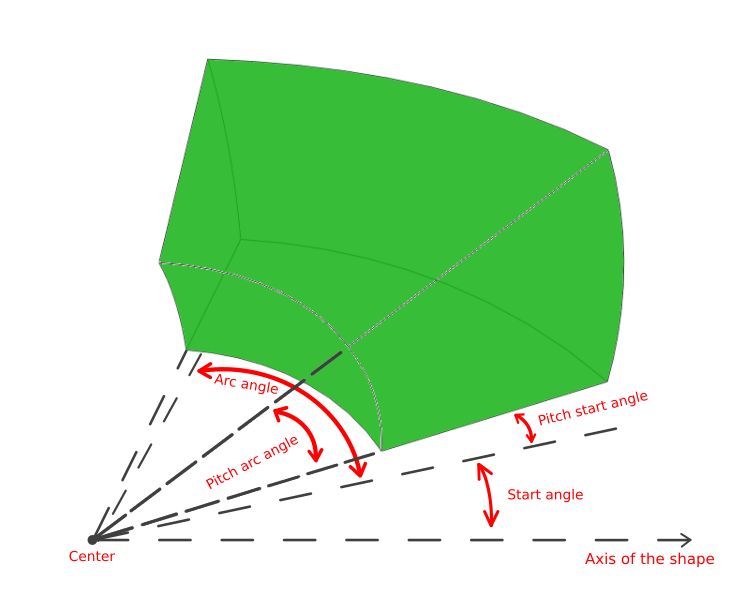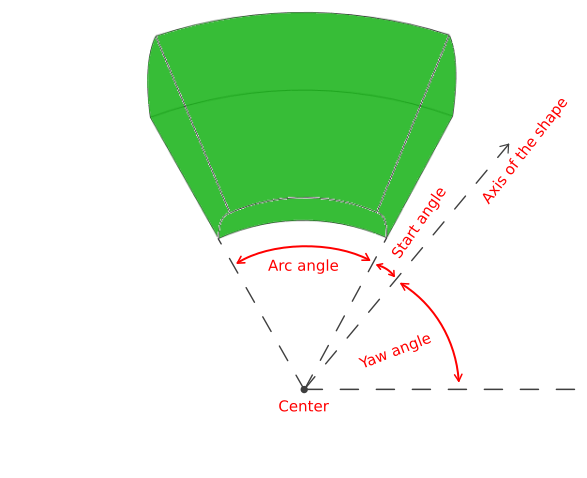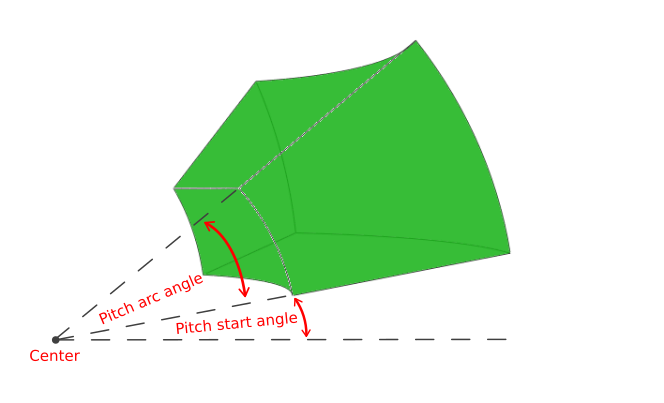2021.0.02

• All Superinterfaces:
java.lang.Cloneable, ILcdBounded, ILcdCloneable, ILcdShape, java.io.Serializable
All Known Subinterfaces:
ILcd3DEditableArcBand
All Known Implementing Classes:
TLcdLonLatHeight3DArcBand, TLcdXYZ3DArcBand

```public interface ILcd3DArcBand
extends ILcdShape```
An `ILcd3DArcBand` is an `ILcdShape` that represents a subvolume of a sphere. The shape extends from a minimum radius to a maximum radius from the center point. It is further delimited by a start angle and an arc angle in one dimension and a pitch start angle and a pitch arc angle in another dimension. The start angle is measured from the 3 o'clock position in the horizontal plane. The pitch start angle is measured from the start angle in the vertical plane.

To allow for orientation, the shape is finally rotated about the yaw axis, followed by a rotation about the pitch axis. These rotations use the axis of the shape as the starting point. This axis is directed towards the 3 o'clock position in the horizontal and in the vertical plane. It is unaffected by start angle, arc angle, pitch start angle and pitch arc angle.

All angles are positive counter-clockwise.Arc angles when axis yaw > 0 Pitch angles

The parameters of the 3D arc band can only be read, not modified. `ILcd3DEditableArcBand` provides an interface to change the parameters.

This shape can, for example, be used to represent a radar coverage in 3D.
Since:
2016.0
• ### Method Summary

All Methods
Modifier and Type Method and Description
`double` `getArcAngle()`
Returns the angle over which this `ILcd3DArcBand` extends (in degrees).
`double` `getAxisPitch()`
Returns the pitch angle of (the axis of) this `ILcd3DArcBand` (in degrees).
`double` `getAxisYaw()`
Returns the yaw angle of (the axis of) this `ILcd3DArcBand` (in degrees).
`ILcdPoint` `getCenter()`
Returns the center point of this `ILcd3DArcBand`.
`double` `getMaxRadius()`
Returns the maximum radius of this `ILcd3DArcBand`.
`double` `getMinRadius()`
Returns the minimum radius of this `ILcd3DArcBand`.
`double` `getPitchArcAngle()`
Returns the angle over which the pitch of this `ILcd3DArcBand` extends (in degrees).
`double` `getPitchStartAngle()`
Returns the start angle of the pitch of this `ILcd3DArcBand` (in degrees).
`double` `getStartAngle()`
Returns the start angle of this `ILcd3DArcBand` (in degrees).
• ### Methods inherited from interface com.luciad.shape.ILcdShape

`contains2D, contains2D, contains3D, contains3D, getFocusPoint`
• ### Methods inherited from interface com.luciad.shape.ILcdBounded

`getBounds`
• ### Methods inherited from interface com.luciad.util.ILcdCloneable

`clone`
• ### Method Detail

• #### getCenter

`ILcdPoint getCenter()`
Returns the center point of this `ILcd3DArcBand`.
Returns:
the center point of this `ILcd3DArcBand`.

`double getMinRadius()`
Returns the minimum radius of this `ILcd3DArcBand`. The minimum radius is smaller than the maximum radius and never smaller than 0.
Returns:
the minimum radius of this `ILcd3DArcBand`.

`double getMaxRadius()`
Returns the maximum radius of this `ILcd3DArcBand`. The maximum radius is larger than the minimum radius and never smaller than 0.
Returns:
the maximum radius of this `ILcd3DArcBand`.
• #### getStartAngle

`double getStartAngle()`
Returns the start angle of this `ILcd3DArcBand` (in degrees). The angle is measured from the axis yaw, positive counter-clockwise.
Returns:
the start angle of this `ILcd3DArcBand` (in degrees). The angle is measured from the axis yaw, positive counter-clockwise.
• #### getArcAngle

`double getArcAngle()`
Returns the angle over which this `ILcd3DArcBand` extends (in degrees). The angle is positive counter-clockwise.
Returns:
the angle over which this `ILcd3DArcBand` extends (in degrees). The angle is positive counter-clockwise.
• #### getPitchStartAngle

`double getPitchStartAngle()`
Returns the start angle of the pitch of this `ILcd3DArcBand` (in degrees). The angle is measured from the axis pitch, positive counter-clockwise.
Returns:
the start angle of the pitch of this `ILcd3DArcBand` (in degrees). The angle is measured from the axis pitch, positive counter-clockwise.
• #### getPitchArcAngle

`double getPitchArcAngle()`
Returns the angle over which the pitch of this `ILcd3DArcBand` extends (in degrees). The angle is positive counter-clockwise.
Returns:
the angle over which the pitch of this `ILcd3DArcBand` extends (in degrees). The angle is positive counter-clockwise.
• #### getAxisYaw

`double getAxisYaw()`
Returns the yaw angle of (the axis of) this `ILcd3DArcBand` (in degrees). The angle is measured from the 3 o'clock position in the horizontal plane, positive counter-clockwise.
Returns:
the yaw angle of (the axis of) this `ILcd3DArcBand` (in degrees). The angle is measured from the 3 o'clock position in the horizontal plane, positive counter-clockwise.
• #### getAxisPitch

`double getAxisPitch()`
Returns the pitch angle of (the axis of) this `ILcd3DArcBand` (in degrees). The angle is measured from the 3 o'clock position in the plane defined by the axis yaw and the vertical axis, positive counter-clockwise.
Returns:
the pitch angle of (the axis of) this `ILcd3DArcBand` (in degrees). The angle is measured from the 3 o'clock position in the plane defined by the axis yaw and the vertical axis, positive counter-clockwise.